## 7.1.1 Problem Formulation

The formulation is designed to allow the tools and concepts learned so far to be applied directly. Let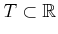denote the time interval, which may be bounded or unbounded. If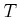is bounded, then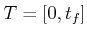, in which 0 is the initial time and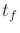is the final time. Ifis unbounded, then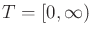. An initial time other than 0 could alternatively be defined without difficulty, but this will not be done here.

Let the state spacebe defined as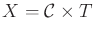, in whichis the usual C-space of the robot, as defined in Chapter 4. A stateis represented as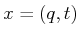, to indicate the configuration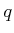and time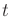components of the state vector. The planning will occur directly in, and in many ways it can be treated as any C-space seen to far, but there is one critical difference: Time marches forward. Imagine a path that travels through. If it first reaches a state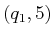, and then later some state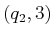, some traveling backward through time is required! There is no mathematical problem with allowing such time travel, but it is not realistic for most applications. Therefore, paths inare forced to follow a constraint that they must move forward in time.

Now consider making the following time-varying versions of the items used in Formulation 4.1 for motion planning.

Formulation 7..1 (The Time-Varying Motion Planning Problem)
1. A world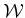in which either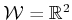or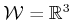. This is the same as in Formulation 4.1.
2. A time intervalthat is either bounded to yieldfor some final time,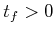, or unbounded to yield.
3. A semi-algebraic, time-varying obstacle region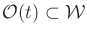for every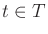. It is assumed that the obstacle region is a finite collection of rigid bodies that undergoes continuous, time-dependent rigid-body transformations.
4. The robot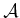(or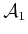,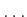,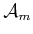for a linkage) and configuration spacedefinitions are the same as in Formulation 4.1.
5. The state spaceis the Cartesian productand a state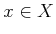is denoted asto denote the configurationand timecomponents. See Figure 7.1. The obstacle region,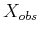, in the state space is defined as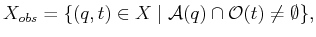(7.1)

and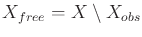. For a given, slices ofand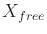are obtained. These are denoted as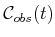and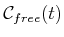, respectively, in which (assumingis one body)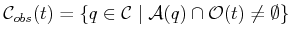(7.2)

and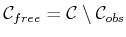.

6. A state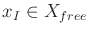is designated as the initial state, with the constraint that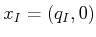for some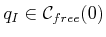. In other words, at the initial time the robot cannot be in collision.
7. A subset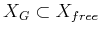is designated as the goal region. A typical definition is to pick some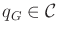and let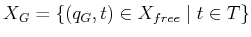, which means that the goal is stationary for all time.
8. A complete algorithm must compute a continuous, time-monotonic path,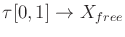, such that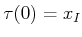and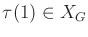, or correctly report that such a path does not exist. To be time-monotonic implies that for any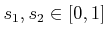such that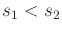, we have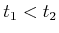, in which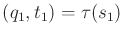and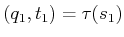.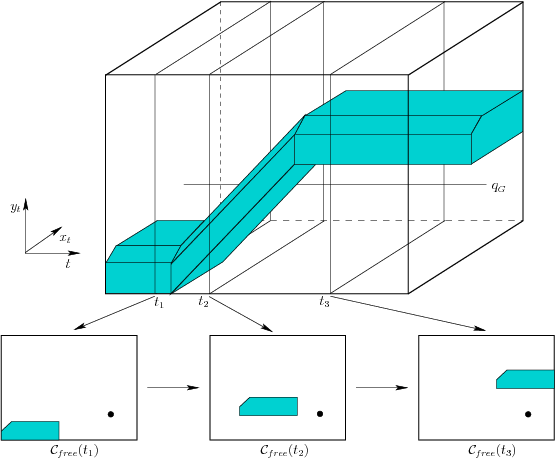Example 7..1 (Piecewise-Linear Obstacle Motion)   Figure 7.1 shows an example of a convex, polygonal robotthat translates in. There is a single, convex, polygonal obstacle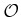. The two of these together yield a convex, polygonal C-space obstacle,, which is shown for times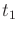,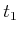, and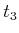. The obstacle moves with a piecewise-linear motion model, which means that transformations applied toare a piecewise-linear function of time. For example, let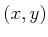be a fixed point on the obstacle. To be a linear motion model, this point must transform as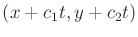for some constants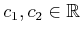. To be piecewise-linear, it may change to a different linear motion at a finite number of critical times. Between these critical times, the motion must remain linear. There are two critical times in the example. Ifis polygonal, and a piecewise-linear motion model is used, thenis polyhedral, as depicted in Figure 7.1. A stationary goal is also shown, which appears as a line that is parallel to the-axis.In the general formulation, there are no additional constraints on the path,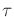, which means that the robot motion model allows infinite acceleration and unbounded speed. The robot velocity may change instantaneously, but the path throughmust always be continuous. These issues did not arise in Chapter 4 because there was no need to mention time. Now it becomes necessary.7.1

Steven M LaValle 2020-08-14